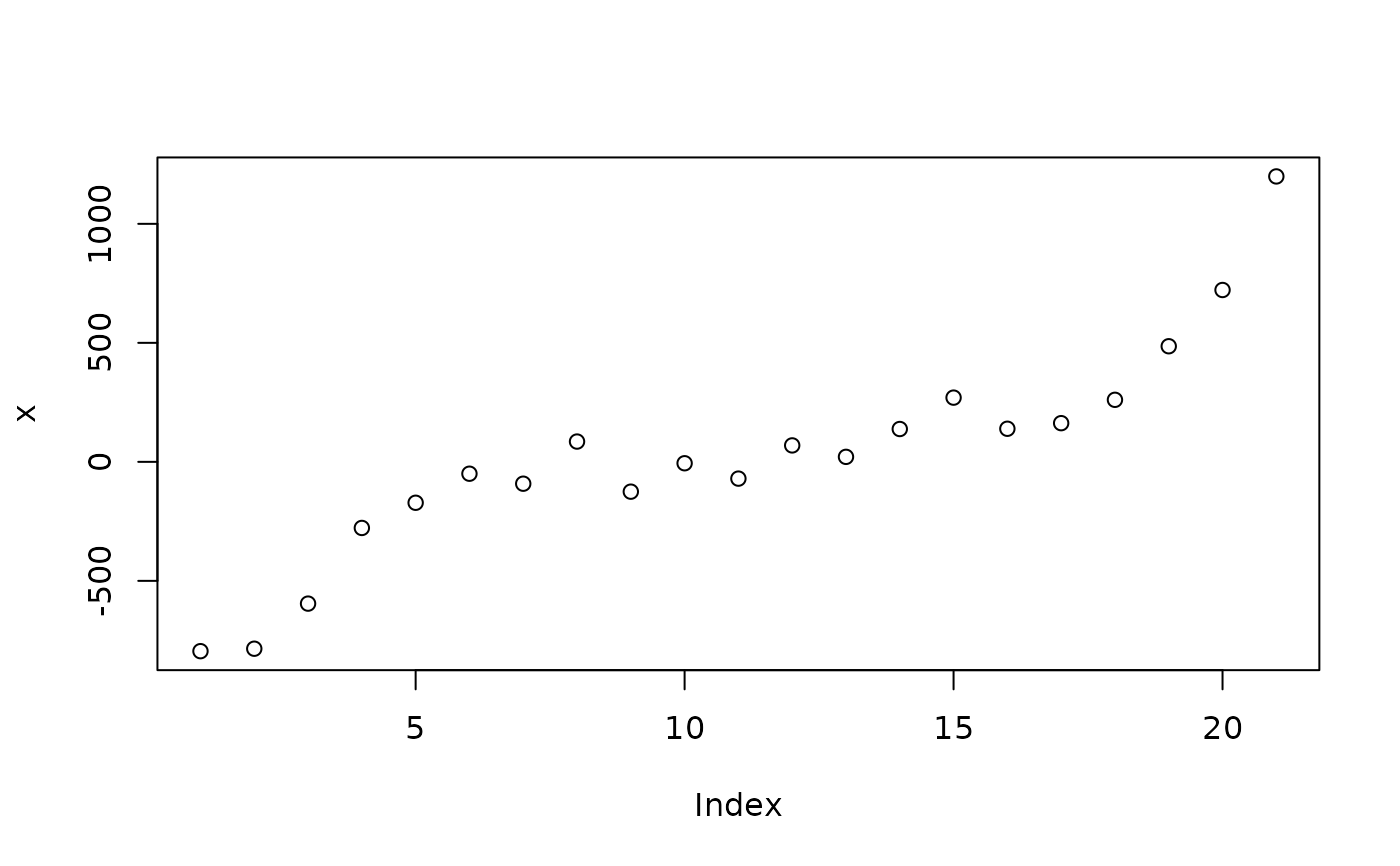Quantify the smoothness of a vector

smoothness(x, method = "cor", lag = 1, iterations = NULL, ...)

## Arguments

x Numeric vector (similar to a time series). Can be "diff" (the standard deviation of the standardized differences) or "cor" (default, lag-one autocorrelation). An integer indicating which lag to use. If less than 1, will be interpreted as expressed in percentage of the length of the vector. The number of bootstrap replicates for computing standard errors. If NULL (default), parametric standard errors are computed. Arguments passed to or from other methods.

## Value

Value of smoothness.

https://stats.stackexchange.com/questions/24607/how-to-measure-smoothness-of-a-time-series-in-r

## Examples

x <- (-10:10)^3 + rnorm(21, 0, 100)
plot(x)smoothness(x, method = "cor")
#>  0.9483709
#> attr(,"class")
#>  "parameters_smoothness" "numeric"
smoothness(x, method = "diff")
#>  1.395158
#> attr(,"class")
#>  "parameters_smoothness" "numeric"